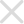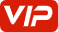## 2019（奕炫GS）• 2019（奕炫GS）
• 维修手册
• 0附录（关于本维修手册使用）
• 如何使用本手册
• 注意事项
• 目录
• 索引
• 10车身装备
• 10.2玻璃、车窗系统和后视镜
• 准备工作
• 初始化
• 前挡风玻璃
• 后三角玻璃
• 后视镜
• 外后视镜拆卸与安装
• 外后视镜电机拆卸与安装
• 外后视镜调节开关拆卸与安装
• 车内后视镜拆卸与安装
• 后风窗玻璃
• 故障诊断
• 注意事项
• 玻璃升降器
• 前车窗升降器开关拆卸与安装
• 前车门玻璃升降器拆卸与安装
• 后车窗升降器开关拆卸与安装
• 后车门玻璃升降器拆卸与安装
• 目录
• 车门玻璃
• 前车门玻璃拆卸与安装
• 后车门玻璃拆卸与安装
• 10.3天窗
• 准备工作
• 天窗
• 天窗总成拆卸与安装
• 天窗排水管拆卸与安装
• 天窗玻璃拆卸与安装
• 天窗电机拆卸与安装
• 天窗系统初始化
• 天窗遮阳板拆卸与安装
• 故障诊断
• 注意事项
• 目录
• 10.5仪表板
• 仪表板
• 仪表板分解
• 仪表板总成分解
• 副仪表板总成分解
• 准备工作
• 副仪表板
• 手套箱
• 故障诊断
• 目录
• 10.6座椅
• 准备工作
• 前排座椅
• 前排座椅前后调节电机的拆卸与安装
• 前排座椅控制面板的拆卸与安装
• 前排座椅的拆卸与安装
• 前排座椅靠背倾角调节电机的拆卸与安装
• 前排座椅高度调节电机总成的拆卸与安装
• 后排座椅
• 后排座椅坐垫的拆卸与安装
• 后排座椅靠背的拆卸与安装
• 注意事项
• 目录
• 11空调系统
• 冷冻油
• 准备工作
• 制冷剂的回收 抽真空 充注 查漏
• 制冷剂的回收 抽真空 充注 （使用充放机）
• 无氟冷媒（R-134a）充放机操作使用说明
• 空调制冷剂量检查
• 空调系统的查漏
• 故障诊断
• 注意事项
• 温度传感器
• 吹脚通风口温度传感器拆装
• 吹脸通风口温度传感器拆装
• 空调蒸发器温度传感器拆卸与安装
• 车内温度传感器拆装
• 车外温度传感器拆装
• 目录
• 空调伺服电机
• 内外循环伺服电机拆卸与安装
• 模式选择伺服电机拆卸与安装
• 温度伺服电机拆卸与安装
• 空调冷凝器
• 空调出风口
• 前排中央出风口拆卸与安装
• 右侧出风口拆卸与安装
• 后出风口拆卸与安装
• 左侧出风口拆卸与安装
• 空调压力开关
• 空调压力开关拆卸与安装
• 空调压力开关拆卸与安装2
• 空调压缩机
• 空调压缩机总成拆卸与安装（A15T+DCT）
• 空调压缩机总成拆卸与安装（C10TD+BE4）
• 空调总成拆卸与安装
• 空调控制单元
• 空调控制面板
• 空调暖风水箱
• 空调滤清器滤芯
• 空调管路组件
• 压缩机高压管拆卸与安装
• 空调高、低压管拆卸与安装
• 空调线束
• 空调膨胀阀
• 空调蒸发器
• 空调通风管
• 空调通风管拆卸与安装（前部）
• 空调通风管拆卸与安装（后部）
• 系统概述
• 维修数据及规格参数
• 阳光传感器
• 鼓风机
• 调速模块拆卸与安装
• 鼓风机总成拆卸与安装
• 12电气系统
• 12.1.起动和充电系统V1
• 准备工作
• 发电机
• 发电机的拆卸与安装(A15T 发动机）
• 发电机的拆卸与安装(C10TDS 发动机）
• 目录
• 系统概述
• 蓄电池
• 蓄电池拆卸与安装（A15T 发动机）
• 蓄电池支架拆卸与安装
• 蓄电池检测与充电
• 起动机
• 起动机的拆卸与安装(A15T 发动机）
• 起动机的拆卸与安装(C10TDS 发动机）
• 12.2智能进入系统
• 一键启动开关
• 一键启动开关的拆卸与安装（A15T+DCT）
• 一键启动开关的拆卸与安装（C10TD+BE4）
• 一键启动开关的拆卸与安装（C10TD+DCT）
• 售后匹配学习
• 售后EMS（A15T）更换匹配
• 售后EMS（C10TD）更换匹配
• 售后IBCM 及EMS 同时更换
• 售后转向柱锁（ESCL）学习
• 售后钥匙学习
• 故障诊断
• 智能进入（PEPS）
• 智能进入收发器拆卸与安装
• 智能进入收发器（副仪表板下部）拆卸与安装
• 智能进入收发器（行李箱处）拆卸与安装
• 智能进入收发器（车门外把手）及车门把手请求开关拆卸与安装
• 注意事项
• 目录
• 系统概述
• 车身控制器（IBCM）
• IBCM 更换后配置写入
• 车身控制器（IBCM）的拆卸与安装
• 12.3照明系统
• 光线雨量传感器
• 准备工作
• 前照灯
• 前照灯总成拆卸与安装
• 调光电机拆卸与安装
• 近光灯灯泡的更换（仅卤素大灯）
• 远光灯灯泡的更换（仅卤素大灯）
• 前雾灯
• 前雾灯总成拆卸与安装
• 前雾灯照射高度手动调节
• 危险报警开关
• 后照灯
• 后组合灯总成（固定侧）拆卸与安装
• 后组合灯总成（移动侧）拆卸与安装
• 后装饰灯总成拆卸与安装
• 后雾灯总成拆卸与安装
• 后雾灯灯泡更换
• 后视镜转向灯
• 故障诊断
• 氛围灯
• 仪表板上氛围灯（右）拆卸与安装
• 仪表板上氛围灯（左）拆卸与安装
• 灯光组合开关
• 牌照灯
• 牌照灯灯泡更换
• 牌照灯的拆卸与安装
• 目录
• 维修数据及规格参数
• 行李箱灯
• 行李箱灯拆卸与安装
• 行李箱灯灯泡更换
• 车灯位置
• 顶灯
• 高位制动灯
• 12.4.驾驶员信息系统V1
• 故障诊断
• 注意事项
• 目录
• 系统概述
• 组合仪表
• 组合仪表的拆卸与安装
• 组合仪表配置写入
• 车辆保养里程重置
• 12.5雨刮
• 光线雨量传感器
• 前雨刮传动机构
• 前雨刮传动机构的拆卸安装
• 前雨刮器刮片更换
• 前雨刮电机拆卸安装
• 前雨刮臂的拆卸与安装
• 喇叭
• 故障诊断
• 注意事项
• 清洗器
• 前清洗器喷嘴拆卸安装
• 洗涤剂储液罐总成拆卸与安装
• 洗涤电机拆卸与安装
• 目录
• 雨刮组合开关
• 12.6车身控制
• 多功能开关
• 故障诊断
• 注意事项
• 目录
• 系统概述
• 车身控制器（IBCM）
• IBCM 更换后配置写入
• 车身控制器（IBCM）的拆卸与安装
• 12.7影音娱乐系统
• 准备工作
• 多媒体影音系统
• MP5 多媒体显示屏拆卸与安装
• MP5 影音主机总成拆卸与安装
• 影音系统配置写入
• 扬声器
• 仪表板上高音扬声器拆卸与安装
• 前门扬声器拆卸与安装
• 后门扬声器拆卸与安装
• 收音机天线
• 故障诊断
• 泊车辅助系统
• 360 环视系统校正
• 倒车雷达控制器拆卸与安装
• 倒车雷达控制器配置写入
• 全景影像控制器拆卸与安装
• 前摄像头拆卸与安装
• 后摄像头拆卸与安装
• 后雷达传感器拆卸与安装
• 电器接口
• 取电接口（副仪表前部）拆卸与安装
• 取电插座的拆卸与安装( 副仪表板后部)
• 目录
• 紧急制动辅助（AEB）雷达传感器
• AEB 雷达传感器拆卸与安装
• AEB 雷达传感器标定升级
• 自适应巡航摄像头
• 环境感知传感器摄像头拆卸与安装
• 环境感知传感器摄像头标定升级
• T-BOX 控制器
• T-BOX 控制器拆卸与安装
• T-BOX 控制器更换后ESK 码写入
• T-BOX 控制器更换后配置写入
• 个人用户注册流程
• 注册激活流程
• 经销商展车注册流程
• 试乘试驾车辆注册流程
• 故障诊断
• 目录
• 12.9CAN网系统
• 故障诊断
• 目录
• 网关控制器
• 网关控制器拆卸与安装
• 网关控制器更换后配置写入
• 1车型介绍
• 主要性能参数
• 主要系统参数
• 产品配置表
• 功能配置写入、匹配及标定说明
• 整车技术参数
• 新功能介绍
• 标识牌位置
• 目录
• 目录索引
• 识别信息
• 车型主要功能差异说明
• 车型分类说明
• 车型配置差异
• 车辆外形尺寸
• 2新车准备
• 东风风神新车准备检查记录表
• 新车准备
• 目录
• 4发动机
• 4.1.发动机结构-A15T
• 准备工作
• 双质量飞轮总成
• 发动机- 变速器总成
• 发动机弹性支架
• 发动机右弹性支架拆卸与安装
• 发动机左弹性支架拆卸与安装
• 抗扭连杆总成拆卸与安装
• 发动机线束
• 变速器分离
• 注意事项
• 目录
• 4.2.发动机润滑-A15T
• 准备工作
• 发动机机油
• 故障诊断
• 油封
• 曲轴前油封拆卸与安装
• 曲轴后油封拆卸与安装
• 油底壳
• 机油集滤器拆卸与安装
• 油底壳拆卸与安装
• 注意事项
• 目录
• 系统概述
• 4.2.发动机润滑系统.-C10TD
• 准备工作
• 发动机机油
• 密封件
• 曲轴前油封拆卸与安装
• 曲轴后油封拆卸与安装
• 故障诊断
• 油底壳
• 注意事项
• 目录
• 系统概述
• 4.3.发动机冷却系统-A15T
• 冷却水泵
• 冷却液
• 冷却风扇
• 冷却风扇拆卸与安装
• 冷却风扇调速模块拆卸与安装
• 准备工作
• 出水室
• 故障诊断
• 散热器
• 散热器进、出水管
• 散热器出水管拆卸与安装
• 散热器进水管拆卸与安装
• 暖风水管
• 暖风水管（金属管）
• 机油冷却器进、出水管
• 机油冷却器出水管拆卸与安装
• 机油冷却器进水管拆卸与安装
• 注意事项
• 涡轮增压器进、出水管
• 目录
• 系统概述
• 维修数据及规格参数
• 膨胀水箱
• 膨胀水箱出水管
• 膨胀水箱通气管
• 节温器
• 4.3.发动机冷却系统-C10TD
• 冷却模块
• 冷却水泵
• 水泵拆卸与安装
• 辅助电动水泵拆卸与安装
• 冷却液
• 冷却管路
• 散热器出水管拆卸与安装
• 散热器进水管拆卸与安装
• 暖风水箱出水管（金属管）拆卸与安装
• 暖风水箱进、出水管（橡胶管）拆卸与安装
• 机油冷却器进、出水管拆卸与安装
• 涡轮增压器进、出水管拆卸与安装
• 冷却风扇
• 冷却风扇拆卸与安装
• 冷却风扇调速模块拆卸与安装
• 准备工作
• 故障诊断
• 散热器
• 注意事项
• 目录
• 系统概述
• 维修数据及规格参数
• 膨胀水箱
• 膨胀水箱出水管
• 膨胀水箱通气管
• 节温器
• 4.4.发动机控制-A15T
• VVT 阀
• 凸轮轴位置传感器
• 加速踏板
• 发动机冷却液温度传感器
• 发动机控制单元
• 发动机爆震传感器
• 售后匹配学习
• 增压压力与温度传感器
• 故障诊断
• 机油压力传感器
• 氧传感器
• 前氧传感器拆卸与安装
• 后氧传感器拆卸与安装
• 注意事项
• 目录
• 碳罐控制阀
• 系统概述
• 转速传感器
• 进气压力传感器
• 进气温度传感器
• 4.5.燃油系统-A15T
• 准备工作
• 故障诊断
• 注意事项
• 燃油喷射机构
• 燃油泵及燃油箱
• 燃油泵拆卸与安装
• 燃油箱总成拆卸与安装
• 燃油管路及滤清器
• 燃油供油管拆卸与安装
• 燃油滤清器拆卸与安装
• 目录
• 碳罐及燃油蒸气管
• 燃油蒸气管拆卸与安装
• 碳罐拆卸与安装
• 碳罐控制阀拆卸与安装
• 碳罐滤清器拆卸与安装
• 碳罐通风电磁阀拆卸与安装
• 系统概述
• 4.5发动机燃油系统-C10TD-DCT
• 准备工作
• 故障诊断
• 注意事项
• 燃油喷射机构
• 喷油器更换
• 喷油器检查
• 燃油分配管拆卸与安装
• 燃油压力传感器拆卸与安装
• 燃油压力检查
• 燃油压力释放
• 燃油泵及燃油箱
• 喷油器更换
• 喷油器检查
• 燃油分配管拆卸与安装
• 燃油压力传感器拆卸与安装
• 燃油压力检查
• 燃油压力释放
• 燃油管路及滤清器
• 燃油供油管拆卸与安装
• 燃油滤清器拆卸与安装
• 目录
• 碳罐及燃油蒸气管
• 燃油蒸气管拆卸与安装
• 碳罐拆卸与安装
• 碳罐控制阀拆卸与安装
• 碳罐滤清器拆卸与安装
• 碳罐通风电磁阀拆卸与安装
• 系统概述
• 高压油泵
• 4.6.进排气系统-A15T
• 中冷器
• 中冷器出气管拆卸与安装
• 中冷器总成拆卸与安装
• 中冷器进气管拆卸与安装
• 准备工作
• 前进气口带进气管总成
• 增压器电子泄压阀
• 增压器电子泄压阀拆卸与安装
• 泄压阀出气管拆卸与安装
• 泄压阀进气管拆卸与安装
• 排气管
• 三元催化器总成拆卸与安装
• 排气歧管拆卸与安装
• 排气管拆卸与安装
• 排气管隔热罩拆卸与安装
• 故障诊断
• 注意事项
• 涡轮增压器
• 增压压力控制阀拆卸与安装
• 增压器进气管拆卸与安装
• 涡轮增压器拆卸与安装
• 电子节气门体
• 目录
• 空气滤清器
• 空气滤清器总成拆卸与安装
• 空气滤清器滤芯拆卸与安装
• 系统概述
• 维修规格及技术参数
• 进气歧管
• 4.6.进排气系统-C10TD
• GPF 积碳处理
• 三元催化器
• 中冷器
• 中冷器出气管拆卸与安装
• 中冷器拆卸与安装
• 中冷器进气管拆卸与安装
• 准备工作
• 压差传感器
• 增压器电子泄压阀
• 增压器电子泄压阀拆卸与安装
• 泄压阀出气管拆卸与安装
• 泄压阀进气管拆卸与安装
• 排气温度传感器
• 排气管
• 排气管总成拆卸与安装
• 排气管隔热罩拆卸与安装
• 故障诊断
• 注意事项
• 涡轮增压器
• 增压压力控制阀拆卸与安装
• 涡轮增压器拆卸与安装
• 涡轮增压器进气管拆卸与安装
• 电子节气门体
• 目录
• 空气滤清器
• 空气滤清器拆卸与安装
• 空气滤清器滤芯拆卸与安装
• 系统概述
• 维修规格及技术参数
• 进气口
• 进气歧管
• 5离合器 变速器
• 5.1.离合器
• 准备工作
• 故障诊断
• 注意事项
• 目录
• 离合器排气
• 离合器检查
• 离合器踏板机构
• 离合器分泵拆卸和安装
• 离合器总成拆卸和安装
• 离合器总泵拆卸和安装
• 离合器踏板拆卸和安装
• 系统概述
• 维修数据及规格
• 5.2.手动变速器
• 准备工作
• 变速器总成
• 变速器控制
• 倒档灯开关拆卸与安装
• 变速器操纵手柄拆卸与安装
• 变速器操纵机构拆卸与安装
• 变速器选、换档拉索拆卸与安装
• 空档传感器拆卸与安装
• 变速器油封
• 变速器右半轴油封拆卸与安装
• 变速器左半轴油封拆卸与安装
• 变速器油液
• 故障诊断
• 注意事项
• 目录
• 系统概述
• 维修数据及规格
• 5.3.双离合变速器
• 传感器
• 压力传感器a 拆卸与安装
• 压力传感器b 拆卸与安装
• 油温传感器拆卸与安装
• 转速传感器拆卸与安装
• 速度传感器拆卸与安装
• 准备工作
• 变速器总成
• 变速器总成拆卸与安装（A15T-DCT）
• 变速器总成拆卸与安装（C10TDS-DCT）
• 变速器油液
• 变速器油液更换
• 变速器驱动泵过滤器拆卸与安装
• 变速器电控单元
• 变速器电控单元拆卸与安装
• 变速器电控单元支架拆卸与安装
• 变速器线束
• 换档控制总成
• 变速器换档执行器拆卸与安装（C10TDS-DCT）
• 换挡手柄及档位装饰板拆卸与安装（A15T-DCT）
• 换档拉索拆卸与安装（A15T-DCT）
• 换档操纵机构拆卸与安装（A15T-DCT）
• 自动换挡器总成拆卸与安装（C10TDS-DCT）
• 选换档执行机构控制器拆卸与安装（C10TDS-DCT）
• 故障诊断
• 油封
• 变速器右半轴油封拆卸与安装
• 变速器左半轴油封拆卸与安装
• 注意事项
• 电机
• 换档电机拆卸与安装
• 离合器冷却油泵电机a 拆卸与安装
• 离合器冷却油泵电机b 拆卸与安装
• 离合器驱动泵电机拆卸与安装
• 目录
• 离合器泵
• 离合器冷却油泵拆卸与安装
• 离合器驱动泵拆卸与安装
• 系统概述
• 维修数据及规格
• 自学习
• 6车桥和悬架
• 6.1前桥与前悬架
• 三角臂
• 准备工作
• 前减震器
• 前减震器总成的分解与安装
• 前减震器总成的拆卸与安装
• 前桥与前悬架
• 前横向稳定杆
• 前横向稳定杆吊耳的拆卸与安装
• 前横向稳定杆的拆卸与安装
• 半轴
• 前半轴总成检查
• 右半轴的拆卸与安装
• 左半轴的拆卸与安装
• 发动机托架
• 故障诊断
• 注意事项
• 目录
• 系统概述
• 维修数据及规格
• 转向节
• 转向节与前轮毂总成分解
• 转向节与前轮毂总成的拆卸与安装
• 6.2后桥与后悬架
• 准备工作
• 后减震器
• 后减震器弹簧的拆卸与安装
• 后减震器的拆卸与安装
• 后托架
• 后悬架左右纵臂衬套拆卸与安装
• 后悬架的拆卸与安装
• 后轮毂及轴承
• 故障诊断
• 注意事项
• 目录
• 系统概述
• 6.3.车轮
• 准备工作
• 四轮定位
• 故障诊断
• 目录
• 系统概述
• 车轮
• 胎压复位
• 车轮的拆卸与安装
• 轮胎的拆卸与安装
• 7制动
• 7.1.制动系统
• 准备工作
• 制动液
• 制动系统组件
• 制动总泵储液罐的拆卸与安装
• 制动总泵的拆卸与安装
• 制动灯开关的拆卸与安装
• 制动踏板的拆卸与安装
• 电子真空泵的拆卸与安装（A15T）
• 电子真空泵的拆卸与安装（C10TD）
• 真空助力器的拆卸与安装
• 前制动系统组件
• 前制动分泵及卡钳的拆卸与安装
• 前制动片的拆卸与安装
• 前制动盘的拆卸与安装
• 前制动软管的拆卸与安装
• 后制动系统组件
• 后制动分泵及卡钳的拆卸与安装
• 后制动片的拆卸与安装
• 后制动盘的拆卸与安装
• 后制动软管的更换
• 故障诊断
• 注意事项
• 目录
• 维修数据与规格参数
• 7.2.驻车制动系统
• 准备工作
• 注意事项
• 目录
• 驻车制动组件
• 后电动驻车马达拆卸与安装
• 电子驻车制动开关拆卸与安装
• 电子驻车制动开关拆卸与安装（A15T）
• 电子驻车制动开关拆卸与安装（C10TD）
• 7.3.制动控制系统
• ESC 液压单元
• ESC 控制单元更换后配置写入
• ESC 液压控制单元的拆卸与安装
• ESC 系统功能标定
• 间接式胎压系统初始化
• 准备工作
• 故障诊断
• 注意事项
• 目录
• 系统概述
• 轮速传感器
• 前轮速传感器的拆卸与安装
• 后轮速传感器的拆卸与安装
• 8转向-8.1.助力转向系统
• 准备工作
• 故障诊断
• 方向盘
• EPS 配置信息
• 方向盘快拨键总成的拆卸与安装
• 方向盘的拆卸与安装
• 旋转开关的拆卸与安装
• 检查与调整
• 注意事项
• 目录
• 维修数据与规格
• 转向器总成
• 横拉杆外球头的拆卸与安装
• 转向器总成的拆卸与安装
• 转向柱总成
• 转向柱锁总成
• 转角自学习
• 9约束系统
• 9.1座椅
• 前排座椅安全带
• 前排座椅安全带带扣的拆卸与安装
• 前排座椅安全带的拆卸与安装
• 后排座椅安全带
• 后排座椅安全带卷收器的拆卸与安装
• 后排座椅安全带带扣的拆卸与安装
• 目录
• 系统概述
• 9.2辅助约束系统
• 准备工作
• 前乘客气囊
• 前座椅气囊
• 故障诊断
• 气囊电控单元
• 注意事项
• 目录
• 碰撞传感器
• 配置写入
• 驾驶员气囊